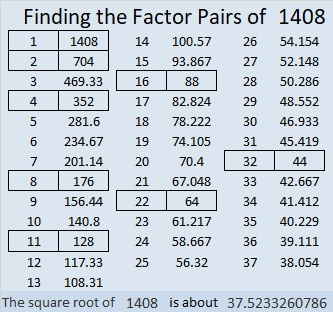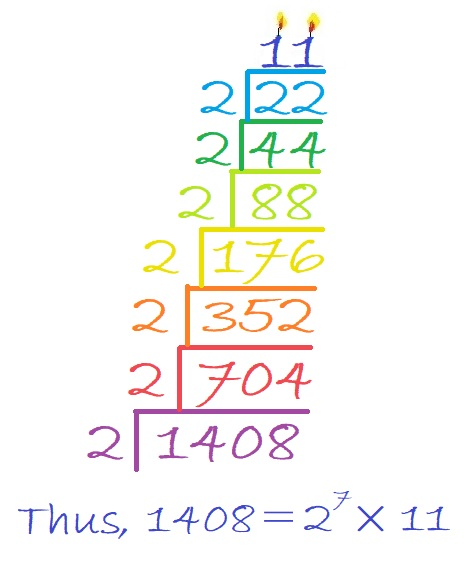# 1408 Powers of 2 in the Multiplication Table

number, puzzle, factors, factor pairs, prime factorization,

I have a 10 × 10 multiplication table poster in my classroom to help students who haven’t memorized the times’ table yet. We have to spend our time going over more advanced topics. One student struggled with the idea of raising two to a power. I went to the poster and boxed in all the powers of two on it. While I boxed them in, I recited, “2⁰ = 1, 2¹ = 2, 2² = 2×2 = 4, 2³ = 2×2×2 = 8, 2⁴ = 2×2×2×2= 16, 2⁵ = 2×2×2×2×2= 32, 2⁶ = 2×2×2×2×2×2=64.”

I liked the pattern those powers of two made on the poster so I made this 32×32 multiplication chart on my computer and continued the pattern.I expect the chart has many things for you to notice and wonder about. You could also do it with powers of 3, or another number, but you would need to use a much bigger multiplication table to show as many powers.

Now I’ll tell you a little bit about the number 1408.

1408 is not a power of 2, but it is 11 times a power of 2, specifically, it is 11 × 2⁷.

• 1408 is a composite number.
• Prime factorization: 1408 = 2 × 2 × 2 × 2 × 2 × 2 × 2 × 11, which can be written 1408 = 2⁷ × 11
• 1408 has at least one exponent greater than 1 in its prime factorization so √1408 can be simplified. Taking the factor pair from the factor pair table below with the largest square number factor, we get √1408 = (√64)(√22) = 8√22
• The exponents in the prime factorization are 7 and 1. Adding one to each exponent and multiplying we get (7 + 1)(1 + 1) = 8 × 2 = 16. Therefore 1408 has exactly 16 factors.
• The factors of 1408 are outlined with their factor pair partners in the graphic below.Here is a festive multilayered factor cake for 1408:So delicious! And here is a nicely balanced factor tree showing all of its prime factors:This site uses Akismet to reduce spam. Learn how your comment data is processed.